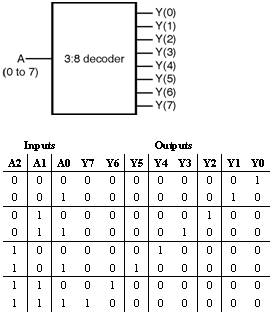Home > VHDL > Logic Circuits > 3 8 Binary Decoder

3:8 Binary Decoder :

All 23 â€“ 8 possible input values of this 3:8 decoder are decoded to a unique output. Figure shows the entity and truthtable of 3:8 Binary Decoder.1) Using case statement :

library IEEE;

use IEEE.STD_LOGIC_1164.all, IEEE.NUMERIC_STD. all;

use IEEE.STD_LOGIC_ARITH.all;

use IEEE.STD_LOGIC_UNSIGNED.all;

entity Decoder3_8 is

Port ( A : in integer range 0 to 7;

Y : out std_logic_vector(7 downto 0));

end Decoder3_8;

architecture behavioral of Decoder3_8 is

begin

process (A)

begin

case A is

when 0 => Y <= "00000001";

when 1 => Y <= "00000010";

when 2 => Y <= "00000100";

when 3 => Y <= "00001000";

when 4 => Y <= "00010000";

when 5 => Y <= "00100000";

when 6 => Y <= "01000000";

when 7 => Y <= "10000000";

end case;

end process ;

end behavioral;

2) Using select signal assignment :

library IEEE;

use IEEE.STD_LOGIC_1164.all, IEEE.NUMERIC_STD. all;

use IEEE.STD_LOGIC_ARITH.all;

use IEEE.STD_LOGIC_UNSIGNED.all;

entity Decoder3_8 is

Port ( A : in integer range 0 to 7;

Y : out std_logic_vector(7 downto 0));

end Decoder3_8;

architecture behavioral of Decoder3_8 is

begin

with A select

Y <= "00000001" when 0,

"00000010" when 1,

"00000100" when 2,

"00001000" when 3,

"00010000" when 4,

"00100000" when 5,

"01000000" when 6,

"10000000" when 7,

"00000000" when others;

end behavioral;

3) Using for loop statement :

library IEEE;

use IEEE.STD_LOGIC_1164.all, IEEE.NUMERIC_STD. all;

use IEEE.STD_LOGIC_ARITH.all;

use IEEE.STD_LOGIC_UNSIGNED.all;

entity Decoder3_8 is

Port ( A : in integer range 0 to 7;

Y : out std_logic_vector(7 downto 0));

end Decoder3_8;

architecture behavioral of Decoder3_8 is

begin

process (A)

begin

for N in 0 to 7 loop

if (A = N) then

Y(N) <= '1';

else

Y(N) <= '0';

end if ;

end loop ;

end process ;

end behavioral;